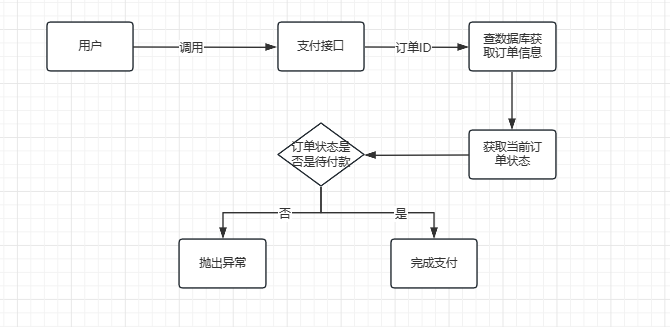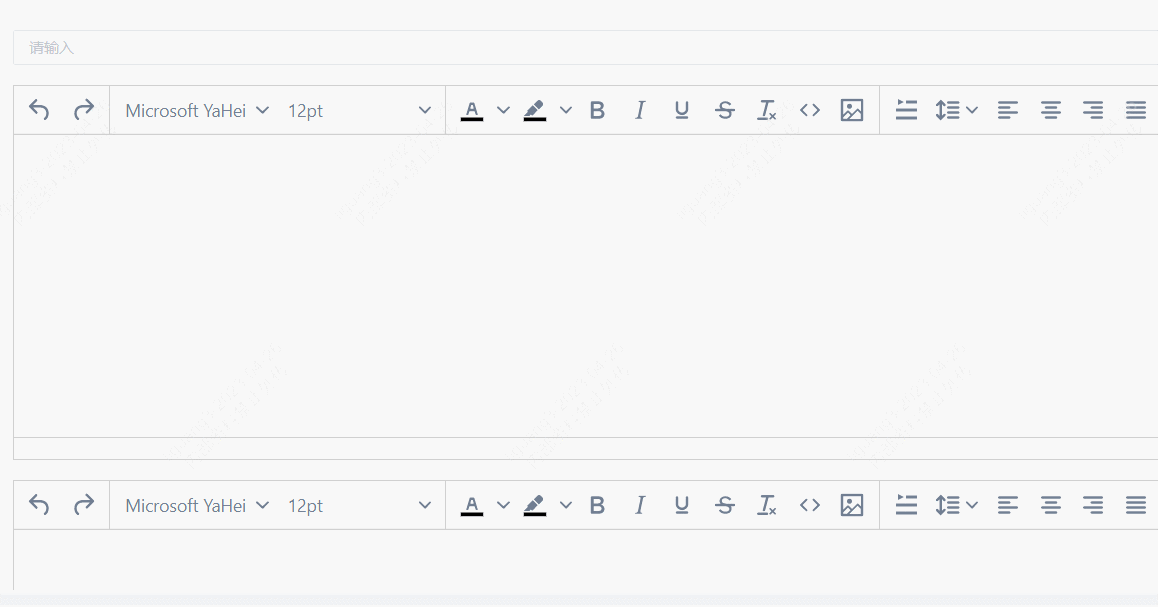# VBA实现Word表格排序

fruit vegetable
apple eggplant
pear carrot
apple cabbage
apple potato
pear yam
orange marrow

（第二列保留其在原始表格中的先后顺序）

fruit vegetable
apple eggplant
apple cabbage
apple potato
pear carrot
pear yam
orange marrow

Sub SortTableInWord()
Dim oTable As Table
Dim i As Long, j As Long
Dim RowsCnt As Long, c As Range
Dim Dic As Object, aKey, aItem, k
Set oTable = ActiveDocument.Tables(1)
RowsCnt = oTable.Rows.Count
ReDim aData(1 To RowsCnt, 1 To 2)
For i = 1 To RowsCnt
For j = 1 To 2
Set c = oTable.Cell(i, j).Range
aData(i, j) = ActiveDocument.Range(c.Start, c.End - 1).Text
Next j
Next i
Set Dic = CreateObject("scripting.dictionary")
For i = 2 To RowsCnt
If Dic.Exists(Temp) Then
Dic(Temp) = Dic(Temp) & "|" & aData(i, 2)
Else
End If
Next i
aKey = Dic.keys
For i = 0 To Dic.Count - 1
For j = i + 1 To Dic.Count - 1
If aKey(i) = aKey(j) Then
Temp = aKey(i)
aKey(i) = aKey(j)
aKey(j) = Temp
End If
Next j
Next i
j = 2
For i = 0 To Dic.Count - 1
aItem = Split(Dic(aKey(i)), "|")
For k = 0 To UBound(aItem)
oTable.Cell(j, 1).Range.Text = aKey(i)
oTable.Cell(j, 2).Range.Text = aItem(k)
j = j + 1
Next
Next i
End Sub


【代码解析】

• 如果存在，则第20行代码将表格中第2列的值追加到字典中，使用竖线作为分隔符。
• 如果不存在，则第22行代码在字典对象中增加新键。